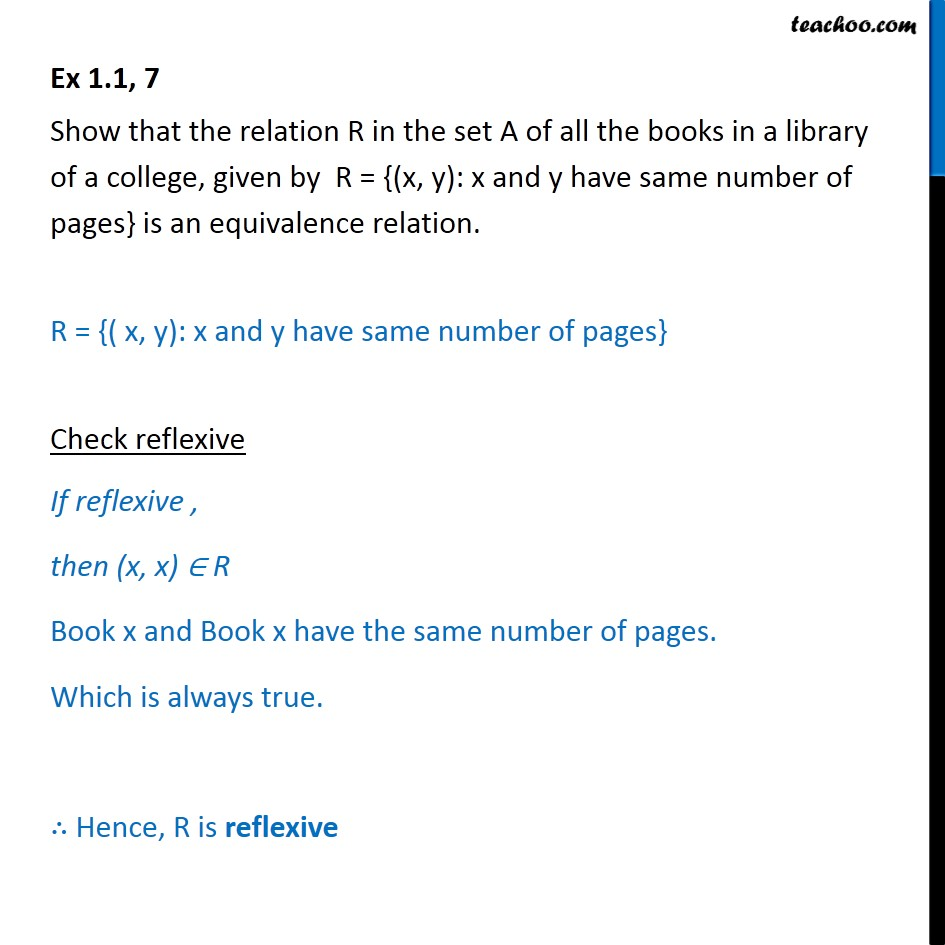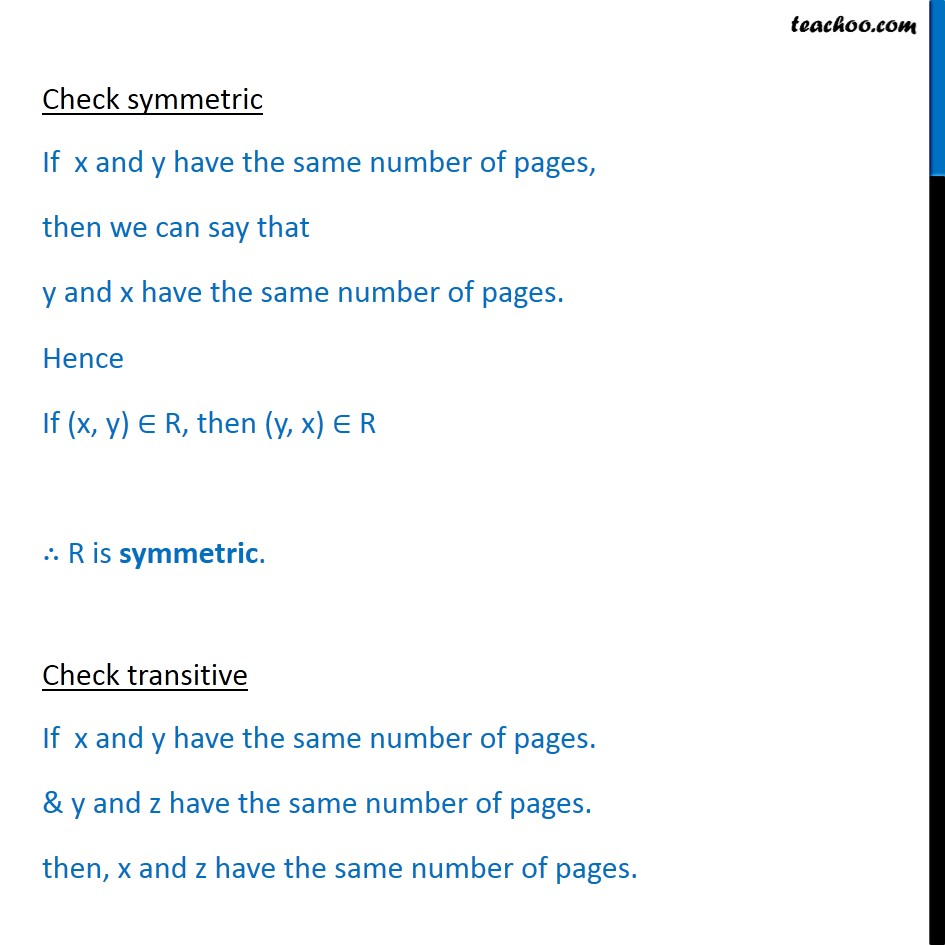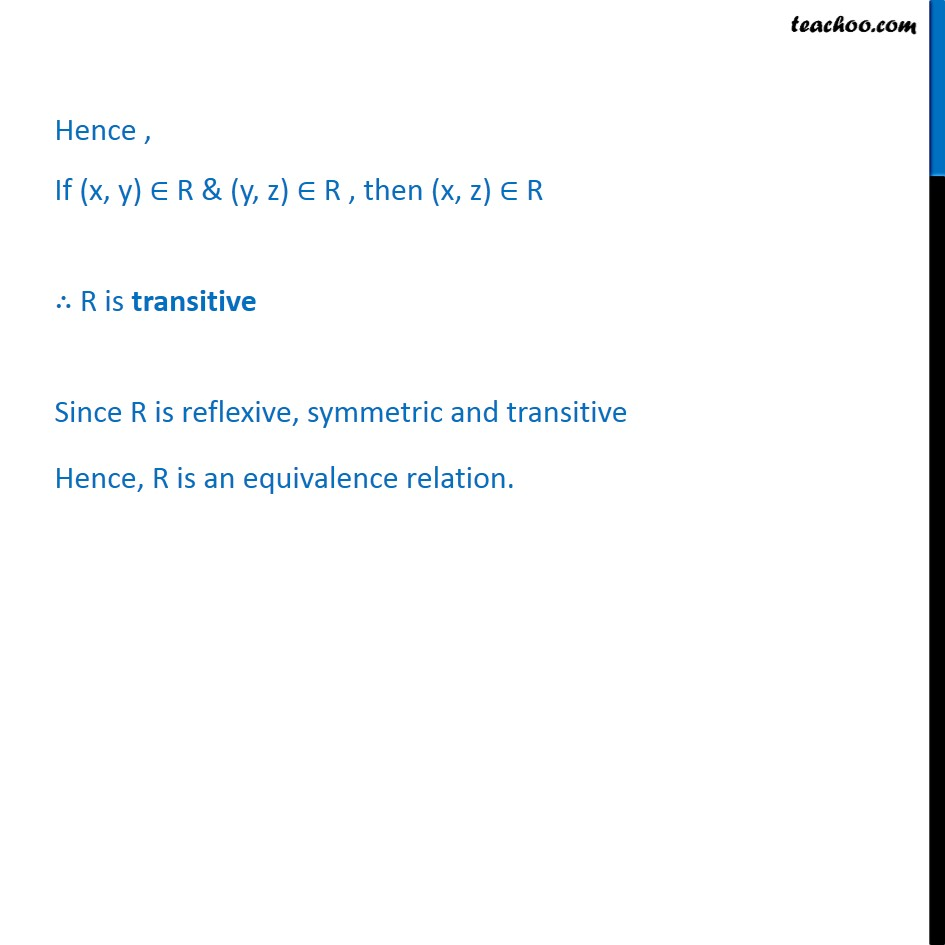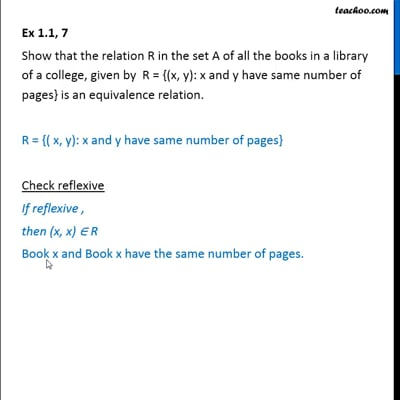To prove relation reflexive, transitive, symmetric and equivalent

Chapter 1 Class 12 Relation and Functions
Concept wiseThis video is only available for Teachoo black users

Introducing your new favourite teacher - Teachoo Black, at only ₹83 per month

### Transcript

Ex 1.1, 7 Show that the relation R in the set A of all the books in a library of a college, given by R = {(x, y): x and y have same number of pages} is an equivalence relation. R = {( x, y): x and y have same number of pages} Check reflexive If reflexive , then (x, x) R Book x and Book x have the same number of pages. Which is always true. Hence, R is reflexive Check symmetric If x and y have the same number of pages, then we can say that y and x have the same number of pages. Hence If (x, y) R, then (y, x) R R is symmetric. Check transitive If x and y have the same number of pages. & y and z have the same number of pages. then, x and z have the same number of pages. Hence , If (x, y) R & (y, z) R , then (x, z) R R is transitive Since R is reflexive, symmetric and transitive Hence, R is an equivalence relation.